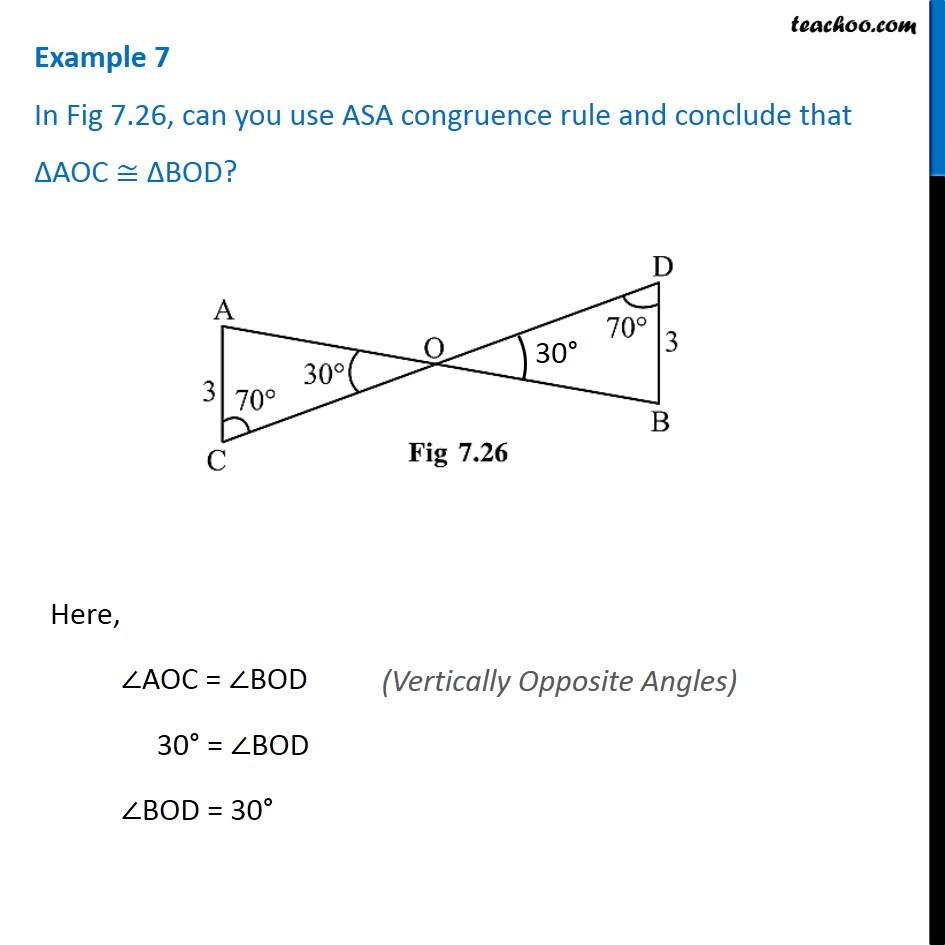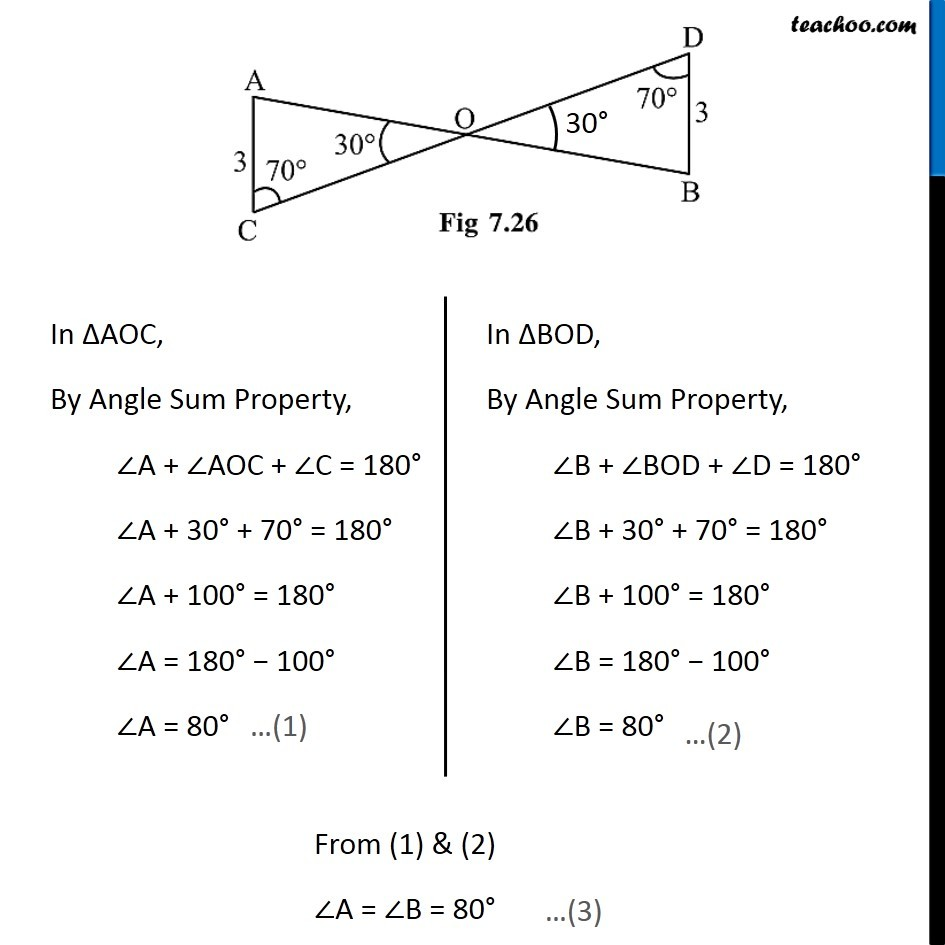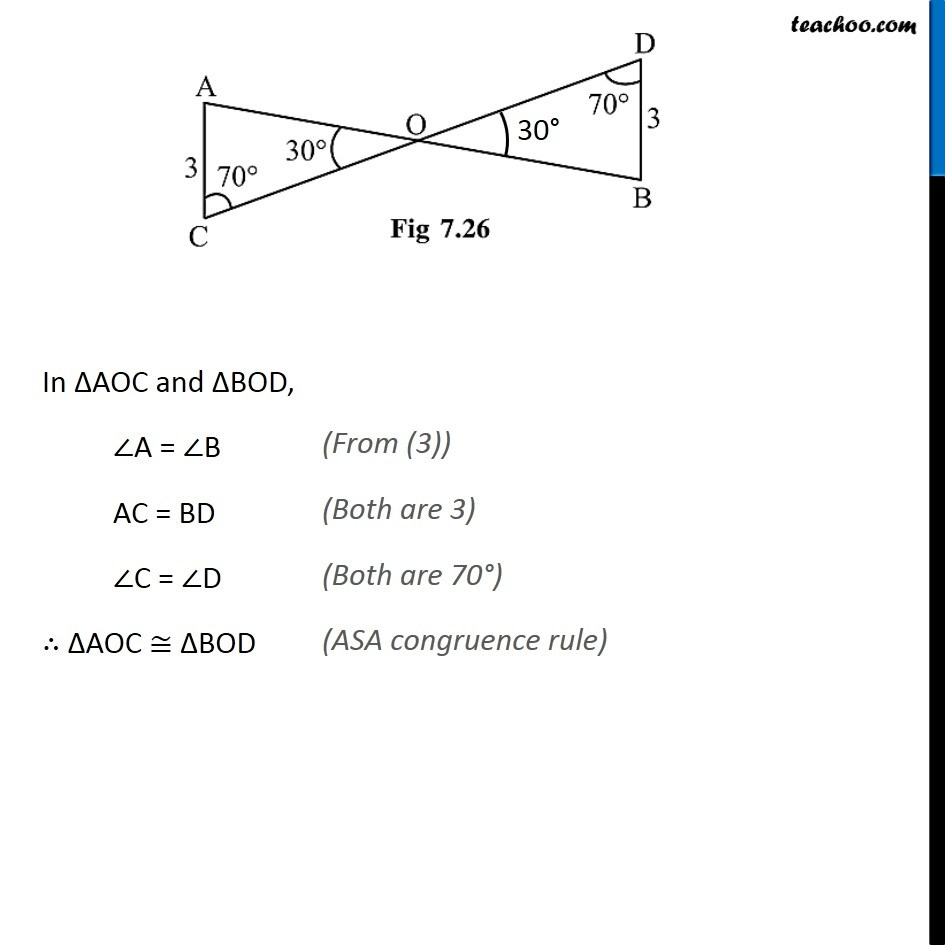1. Chapter 7 Class 7 Congruence of Triangles
2. Concept wise
3. ASA Congruency Criteria

Transcript

Example 7 In Fig 7.26, can you use ASA congruence rule and conclude that ∆AOC ≅ ∆BOD?Here, ∠AOC = ∠BOD 30° = ∠BOD ∠BOD = 30° In ∆AOC, By Angle Sum Property, ∠A + ∠AOC + ∠C = 180° ∠A + 30° + 70° = 180° ∠A + 100° = 180° ∠A = 180° − 100° ∠A = 80° In ∆BOD, By Angle Sum Property, ∠B + ∠BOD + ∠D = 180° ∠B + 30° + 70° = 180° ∠B + 100° = 180° ∠B = 180° − 100° ∠B = 80° From (1) & (2) ∠A = ∠B = 80° In ∆AOC and ∆BOD, ∠A = ∠B AC = BD ∠C = ∠D ∴ ∆AOC ≅ ∆BOD

ASA Congruency Criteria